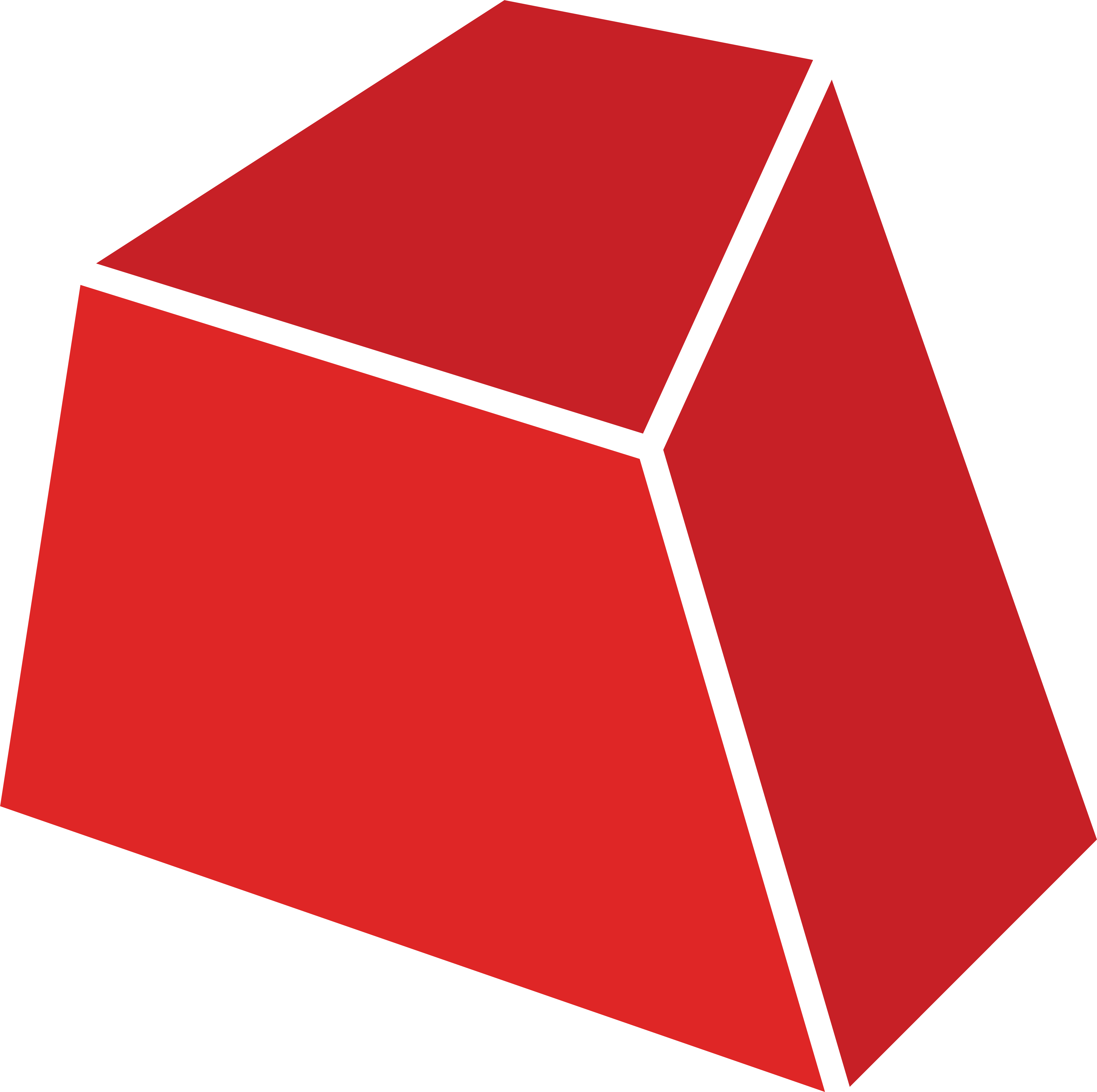#Gurobi.Optimizer 10.0.1.4Prefix Reserved
.NET Standard 2.0

Requires NuGet 3.3.0 or higher.

`dotnet add package Gurobi.Optimizer --version 10.0.1.4`
`NuGet\Install-Package Gurobi.Optimizer -Version 10.0.1.4`
This command is intended to be used within the Package Manager Console in Visual Studio, as it uses the NuGet module's version of Install-Package.
`<PackageReference Include="Gurobi.Optimizer" Version="10.0.1.4" />`
For projects that support PackageReference, copy this XML node into the project file to reference the package.
`paket add Gurobi.Optimizer --version 10.0.1.4`
`#r "nuget: Gurobi.Optimizer, 10.0.1.4"`
#r directive can be used in F# Interactive and Polyglot Notebooks. Copy this into the interactive tool or source code of the script to reference the package.
```// Install Gurobi.Optimizer as a Cake Addin

// Install Gurobi.Optimizer as a Cake Tool
#tool nuget:?package=Gurobi.Optimizer&version=10.0.1.4```

## Gurobi Optimizer

This package contains the Gurobi Optimizer v10.0.2. It is compatible with .NET Standard 2.0 or higher.

For an easier start with Gurobi's .NET API, we provide an introduction to the .NET Interface (C#) in Gurobi and multiple .NET code examples showing most of Gurobi's features. You can find all information on methods available in the Gurobi's .NET API in our documentation.

For more information on installing the Gurobi NuGet package please refer to one of our Quickstart Guides:

If you encounter any issues during the installation process and could not find an appropriate answer in Gurobi's Knowledge Base, please open a Support Request through our Support Portal.

The following example formulates and solves a simple MIP model via Gurobi's .NET interface.

``````/*
maximize    x +   y + 2 z
subject to  x + 2 y + 3 z <= 4
x +   y       >= 1
x, y, z binary
*/

using System;
using Gurobi;

class mip1_cs
{
static void Main()
{
// Create an empty environment, set options and start
GRBEnv env = new GRBEnv(true);
env.Set("LogFile", "mip1.log");
env.Start();

// Create empty model
GRBModel model = new GRBModel(env);

// Create variables
GRBVar x = model.AddVar(0.0, 1.0, 0.0, GRB.BINARY, "x");
GRBVar y = model.AddVar(0.0, 1.0, 0.0, GRB.BINARY, "y");
GRBVar z = model.AddVar(0.0, 1.0, 0.0, GRB.BINARY, "z");

// Set objective: maximize x + y + 2 z
model.SetObjective(x + y + 2 * z, GRB.MAXIMIZE);

// Add constraint: x + 2 y + 3 z <= 4
model.AddConstr(x + 2 * y + 3 * z <= 4.0, "c0");

// Add constraint: x + y >= 1
model.AddConstr(x + y >= 1.0, "c1");

// Optimize model
model.Optimize();

Console.WriteLine(x.VarName + " " + x.X);
Console.WriteLine(y.VarName + " " + y.X);
Console.WriteLine(z.VarName + " " + z.X);

Console.WriteLine("Obj: " + model.ObjVal);

// Dispose of model and env
model.Dispose();
env.Dispose();
}
}
``````
Product Compatible and additional computed target framework versions.
.NET net5.0 was computed.  net5.0-windows was computed.  net6.0 was computed.  net6.0-android was computed.  net6.0-ios was computed.  net6.0-maccatalyst was computed.  net6.0-macos was computed.  net6.0-tvos was computed.  net6.0-windows was computed.  net7.0 was computed.  net7.0-android was computed.  net7.0-ios was computed.  net7.0-maccatalyst was computed.  net7.0-macos was computed.  net7.0-tvos was computed.  net7.0-windows was computed.
.NET Core netcoreapp2.0 was computed.  netcoreapp2.1 was computed.  netcoreapp2.2 was computed.  netcoreapp3.0 was computed.  netcoreapp3.1 was computed.
.NET Standard netstandard2.0 is compatible.  netstandard2.1 was computed.
.NET Framework net461 was computed.  net462 was computed.  net463 was computed.  net47 was computed.  net471 was computed.  net472 was computed.  net48 was computed.  net481 was computed.
MonoAndroid monoandroid was computed.
MonoMac monomac was computed.
MonoTouch monotouch was computed.
Tizen tizen40 was computed.  tizen60 was computed.
Xamarin.iOS xamarinios was computed.
Xamarin.Mac xamarinmac was computed.
Xamarin.TVOS xamarintvos was computed.
Xamarin.WatchOS xamarinwatchos was computed.
Compatible target framework(s)
• #### .NETStandard 2.0

• No dependencies.

### NuGet packages (1)

Showing the top 1 NuGet packages that depend on Gurobi.Optimizer:

OPTANO.Modeling.Gurobi